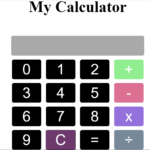## Functional Calculator In JavaScript With Source Code

The Functional calculator is a simple project in HTML5, CSS, and JavaScript. Here, the calculator functions as that of a real-life simple calculator. This project is for solving the mathematical calculations of numbers. You can see the basic features of t...

Type : Project

File Size : 968 bytes# Similar Projects and Reports

#### Functional Calculator In JavaScript With Source Code

The Functional calculator is a simple project in HTML5, CSS, and JavaScript. Here, the calculator functions as that of a real-life simple calculator. This project is for solving the mathematical calculations of numbers. You can see the basic features of t...

#### Calculator In JavaScript With Source Code

The calculator is a simple project in HTML5, CSS, and JavaScript. Here, the calculator functions as that of a real-life simple calculator. This project is for solving the mathematical calculations of numbers. You can see the basic features of the calculat...

#### Web Calculator In JavaScript with Source Code

Web Calculator is a simple project in HTML5, CSS, and JavaScript. This project is for solving the mathematical calculations of numbers. You can see the basic features of the advanced calculator in this project. Also, there are two modes in this calculator...

#### BMI CALCULATOR IN JAVASCRIPT WITH SOURCE CODE

BMI Calculator is a simple project developed using HTML5, CSS, and JavaScript. This project is for checking your BMI. With the BMI value, you can check whether you have a healthy weight or not. Also, you can see the basic features of this calculator in th...

#### Tip Calculator In Javascript With Source Code

The Tip Calculator is a simple project developed using JavaScript, CSS, and HTML. This project is an interesting project. The user can calculate the tip amount that they can get from a single bill pay.

#### JavaScript Calculator With Source Code

This javascript calculator project is a simple project in HTML5, CSS, and JavaScript. Here, the calculator functions as that of a real-life simple calculator. This project is for solving the mathematical calculations of numbers. You can see the basic feat...